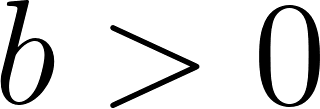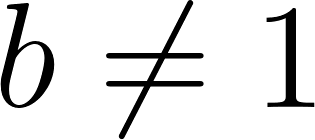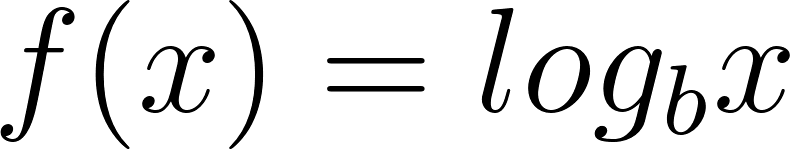×
Get Full Access to Calculus: Early Transcendentals - 1 Edition - Chapter 1.3 - Problem 9e
Get Full Access to Calculus: Early Transcendentals - 1 Edition - Chapter 1.3 - Problem 9e

×

# For b > 0 with b 1, what are the domain and rang e of f(xISBN: 9780321570567 2

## Solution for problem 9E Chapter 1.3

Calculus: Early Transcendentals | 1st Edition

• Textbook Solutions
• 2901 Step-by-step solutions solved by professors and subject experts
• Get 24/7 help from StudySoup virtual teaching assistantsCalculus: Early Transcendentals | 1st Edition

4 5 1 381 Reviews
21
5
Problem 9E

Forwith, what is the domain and range ofand why?

Step-by-Step Solution:

Step 1 of 4

Given,We need to find the domain and range of the given function forwith.

Step 2 of 4

Step 3 of 4

##### ISBN: 9780321570567

The answer to “?For with , what is the domain and range of and why?” is broken down into a number of easy to follow steps, and 12 words. This textbook survival guide was created for the textbook: Calculus: Early Transcendentals, edition: 1. Calculus: Early Transcendentals was written by and is associated to the ISBN: 9780321570567. Since the solution to 9E from 1.3 chapter was answered, more than 315 students have viewed the full step-by-step answer. This full solution covers the following key subjects: domain, log, rang. This expansive textbook survival guide covers 85 chapters, and 5218 solutions. The full step-by-step solution to problem: 9E from chapter: 1.3 was answered by , our top Calculus solution expert on 03/03/17, 03:45PM.

Unlock Textbook Solution